## Figures index

#### Bonini Neto A., Alves D. A.

World Journal Control Science and Engineering. 2014, 2(1), 18-24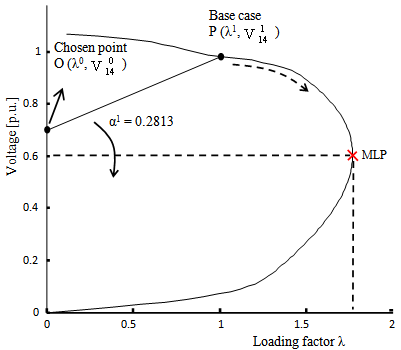• Figure 1. Performance of the method for the IEEE 14-bus system: first line through a chosen point O (λ0, V0) in the λV plan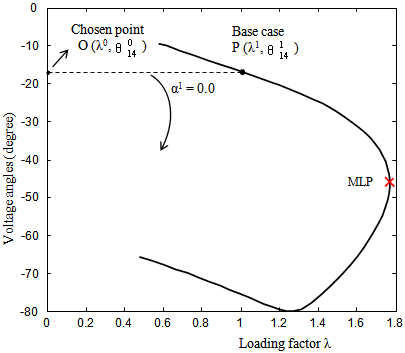• Figure 2. Performance of the method for the IEEE 14-bus system: first line through a chosen point O (λ0, θ0) in the λV plan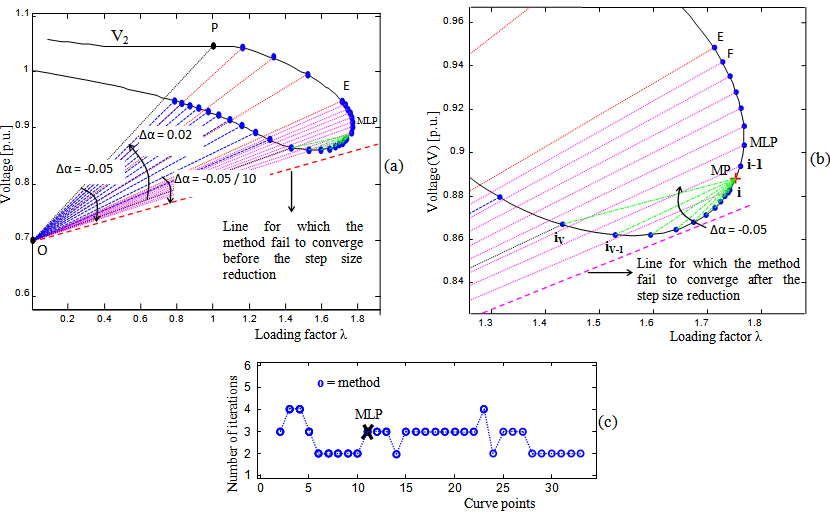• Figure 3. Performance of the method for the IEEE 14-bus system: (a) voltage magnitude at the generation bus 2 (V2) as function of λ, (b) detail of the MLP region, (c) number of iterations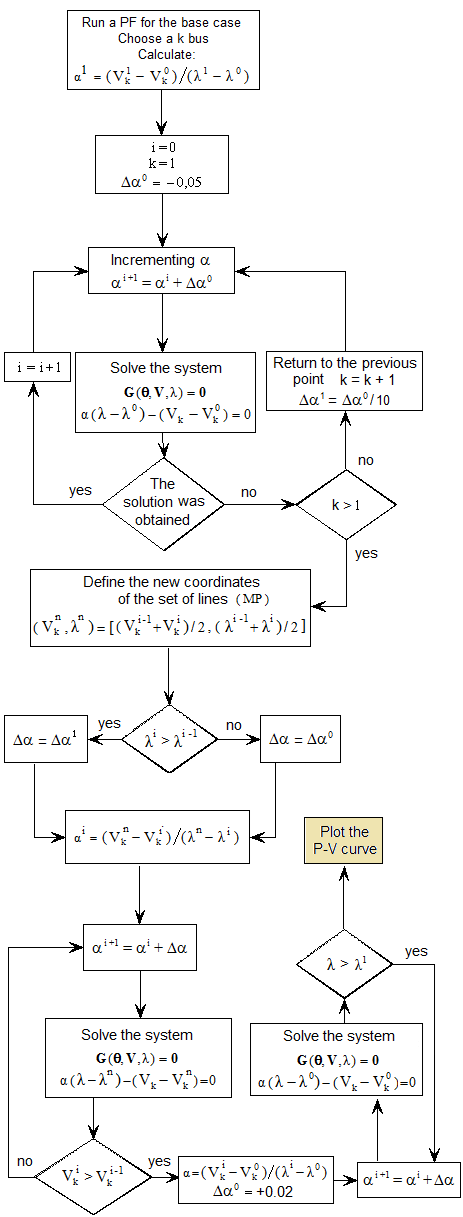• Figure 4. Flowchart of the method using the λV plan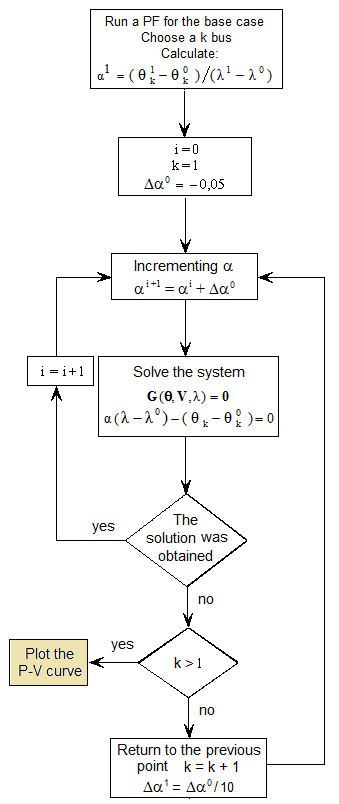• Figure 5. Flowchart of the method using the λ-θ plan• Figure 6. Performance of the proposed method for the IEEE 14-bus system: (a) voltage angle at the critical bus 14 (V14) as function of λ, (b) points of P-V curve stored by the proposed method, (c) number of iterations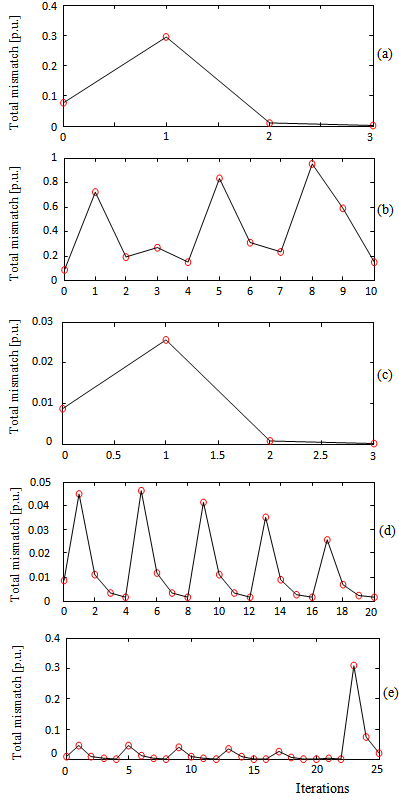• Figure 7. Convergence patterns of the PCPF at different P-V curve points: (a) for point "E", (b) for the next point of point "E" and keeping the step size of -0,05, (c) for point "b" after a step size reduction, (d) for the next point of point "b" with a maximum number of iterations of 20, (e) for the next point of point "b" with a maximum number of iterations of 25